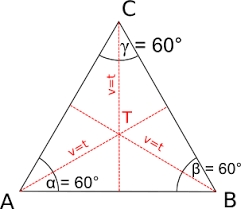# Three parallels

The vertices of an equilateral triangle lie on 3 different parallel lines. The middle line is 5 m and 3 m distant from the end lines. Calculate the height of this triangle.

Result

v =  7 m

#### Solution:

$a^2 = 3^2 +x^2 \ \\ a^2 = 5^2 + y^2 \ \\ a^2 = 8^2 + (y-x)^2 \ \\ \ \\ 3^2 +x^2 = 5^2 + y^2 \ \\ 3^2 +x^2 = 8^2 + (y-x)^2 \ \\ \ \\ 3^2 +x^2 = 8^2 + y^2-2xy +x^2 \ \\ 3^2 = 8^2 + y^2-2xy \ \\ \ \\ x = (8^2 - 3^2 + y^2) / (2y) \ \\ \ \\ y = 11 / \sqrt{ 3 } \doteq 6.3509 \ m \ \\ \ \\ x = (8^2 - 3^2 + y^2) / (2y) = (8^2 - 3^2 + 6.3509^2) / (2 \cdot \ 6.3509) \doteq 7.5056 \ m \ \\ \ \\ a = \sqrt{ 3^2 + x^2 } = \sqrt{ 3^2 + 7.5056^2 } \doteq 8.0829 \ m \ \\ \ \\ v^2 = a^2 - (a/2)^2 \ \\ \ \\ v = \sqrt{ a^2 - (a/2)^2 } = \sqrt{ 8.0829^2 - (8.0829/2)^2 } = 7 = 7 \ \text { m }$Our examples were largely sent or created by pupils and students themselves. Therefore, we would be pleased if you could send us any errors you found, spelling mistakes, or rephasing the example. Thank you!

Leave us a comment of this math problem and its solution (i.e. if it is still somewhat unclear...):Be the first to comment!#### Following knowledge from mathematics are needed to solve this word math problem:

Looking for help with calculating roots of a quadratic equation? Do you have a linear equation or system of equations and looking for its solution? Or do you have quadratic equation? Do you want to convert length units? Pythagorean theorem is the base for the right triangle calculator. See also our trigonometric triangle calculator.

## Next similar math problems:Show that the quadrilateral with vertices P1(0,1), P2(4,2) P3(3,6) P4(-5,4) has two right triangles.
2. Trapezoid MO-5-Z8ABCD is a trapezoid that lime segment CE divided into a triangle and parallelogram as shown. Point F is the midpoint of CE, DF line passes through the center of the segment BE and the area of the triangle CDE is 3 cm2. Determine the area of the trapezoid A
3. Trapezoid MOThe rectangular trapezoid ABCD with right angle at point B, |AC| = 12, |CD| = 8, diagonals are perpendicular to each other. Calculate the perimeter and area of ​​the trapezoid.
4. RectangleIn rectangle with sides, 6 and 3 mark the diagonal. What is the probability that a randomly selected point within the rectangle is closer to the diagonal than to any side of the rectangle?
5. 30-gonAt a regular 30-gon the radius of the inscribed circle is 15cm. Find the "a" side size, circle radius "R", circumference, and content area.
6. Quarter circleWhat is the radius of a circle inscribed in the quarter circle with a radius of 100 cm?
7. Ratio of sidesCalculate the area of a circle that has the same circumference as the circumference of the rectangle inscribed with a circle with a radius of r 9 cm so that its sides are in ratio 2 to 7.
8. Annular areaThe square with side a = 1 is inscribed and circumscribed by circles. Find the annular area.
9. The trapeziumThe trapezium is formed by cutting the top of the right-angled isosceles triangle. The base of the trapezium is 10 cm and the top is 5 cm. Find the area of trapezium.
10. Area of a rectangleCalculate the area of a rectangle with a diagonal of u = 12.5cm and a width of b = 3.5cm. Use the Pythagorean theorem.
11. GardenArea of a square garden is 6/4 of triangle garden with sides 56 m, 35 m, and 35 m. How many meters of fencing need to fence a square garden?
12. RectangleThe rectangle is 21 cm long and 38 cm wide. Determine the radius of the circle circumscribing rectangle.
13. Triangle SASCalculate the area and perimeter of the triangle, if the two sides are 51 cm and 110 cm long and angle them clamped is 130 °.
14. RhombusCalculate the perimeter and area of ​​a rhombus whose diagonals are 39 cm and 51 cm long.
15. Square diagonalCalculate the length of the square diagonal if the perimeter is 476 cm.
16. RhombusIt is given a rhombus of side length a = 29 cm. Touch points of inscribed circle divided his sides into sections a1 = 14 cm and a2 = 15 cm. Calculate the radius r of the circle and the length of the diagonals of the rhombus.
17. Circular poolThe base of the pool is a circle with a radius r = 10 m, excluding a circular segment that determines the chord length 10 meters. The pool depth is h = 2m. How many hectoliters of water can fit into the pool?